# Decagon

A polygon with 10 sides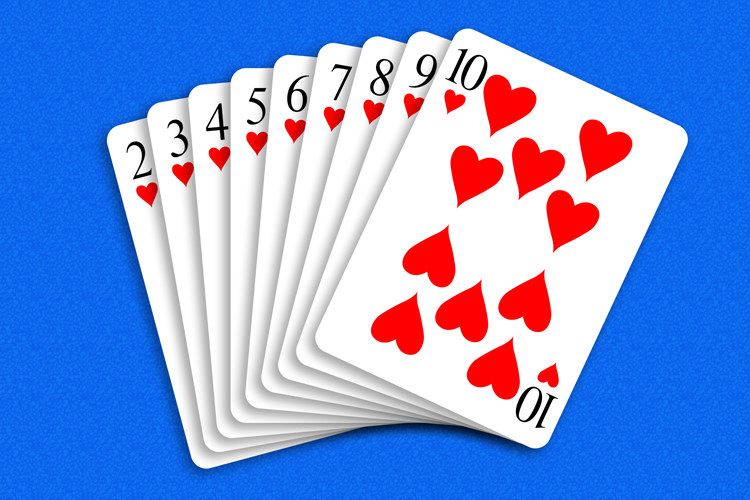In a deck of cards the highest number is a 10 (Dec has the same word root as decade which is 10 years).

Example 1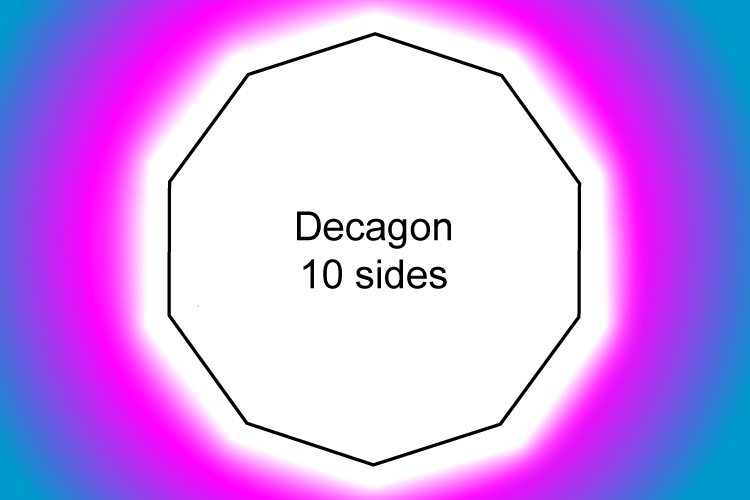All 10 sides of a regular decagon are equal in length.

Example 2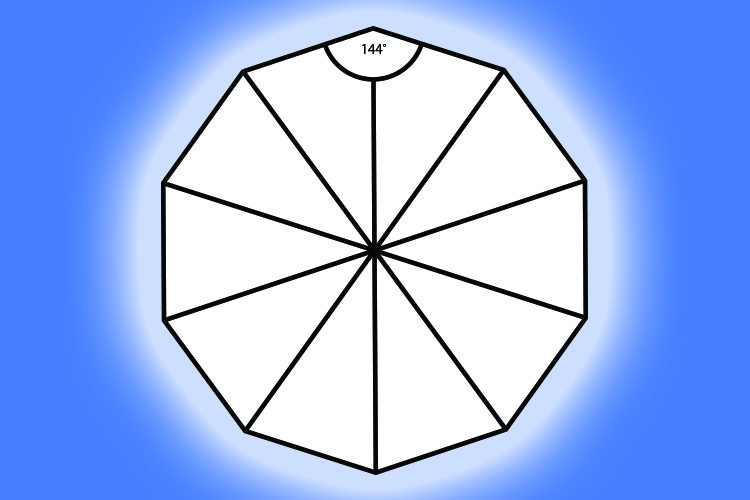The internal angle between the sides of a regular decagon is 144°.

Example 3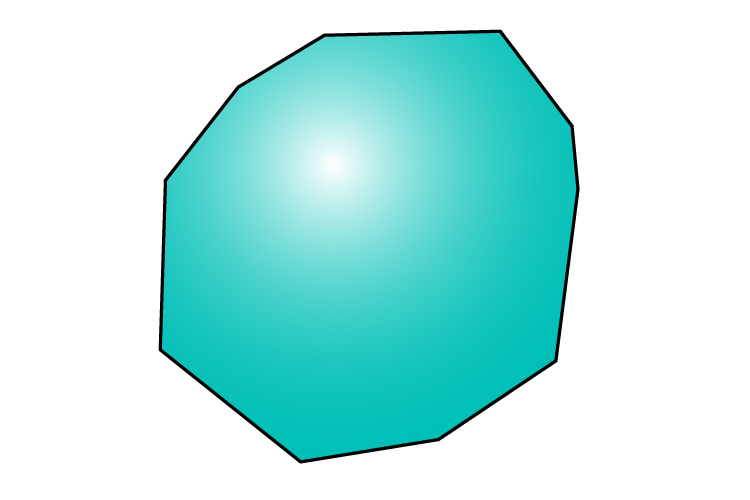An irregular decagon such as the one above may be convex as in this case.

Example 4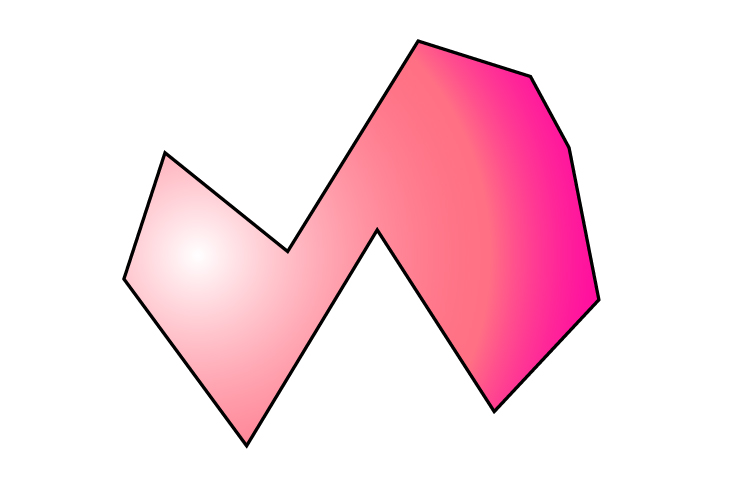The decagon above is an irregular concave decagon.

Example 5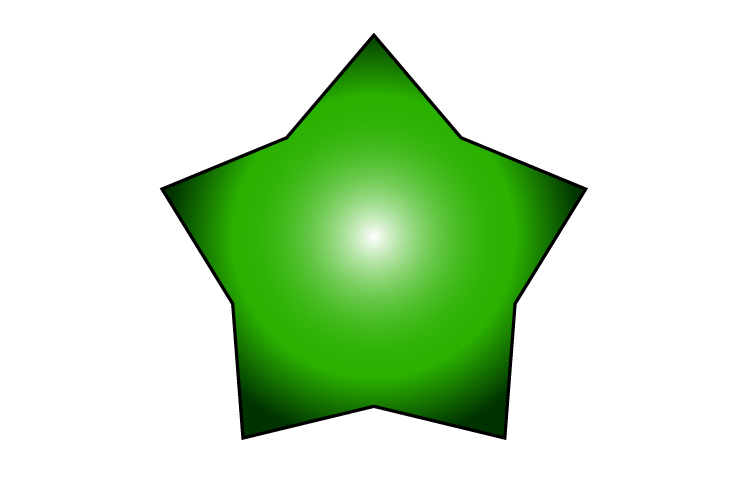This star shape is an irregular decagon as the internal angles between the sides are not all equal.